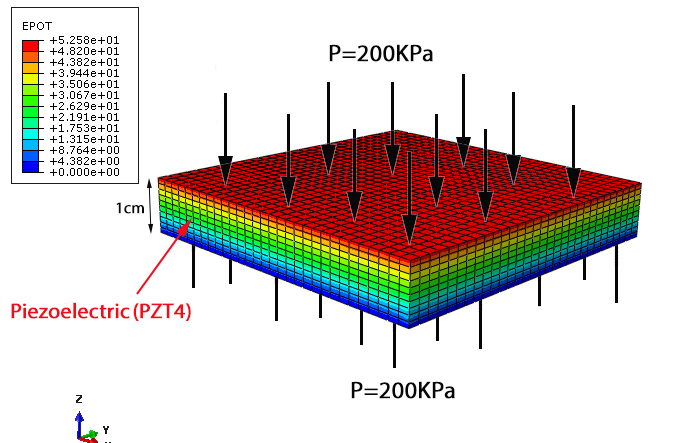Sale!

0

Simulation the Piezoelectric under a uniform pressure Abaqus

Download link includes full video (step by step) – Cae file – inp file – Excel file

This tutorial is free

## Description

Simulation the Piezoelectric under a uniform pressure Abaqus

In this example, we intend to simulate the Piezoelectric under a uniform pressure in the Abaqus software. The Piezoelectric that is used in this question is PZT4 and we intend to apply a pressure of 200 KPa to the Piezoelectric and calculate the Output voltage using the Abaqus softwareA uniform pressure will be applied to the top and bottom surfaces of Piezoelectric

a 200 KPa pressure has been applied to the top and bottom surfaces of the Piezoelectric that causes an electric potential difference to be created in the Piezoelectric , and we intend to calculate this electric potential difference

As you see , if we apply a uniform pressure equal to 200 KPa to the Piezoelectric , a voltage of 50 Volts will be created in Piezoelectric. in other words , piezoelectric output voltage is equal to 50 volts